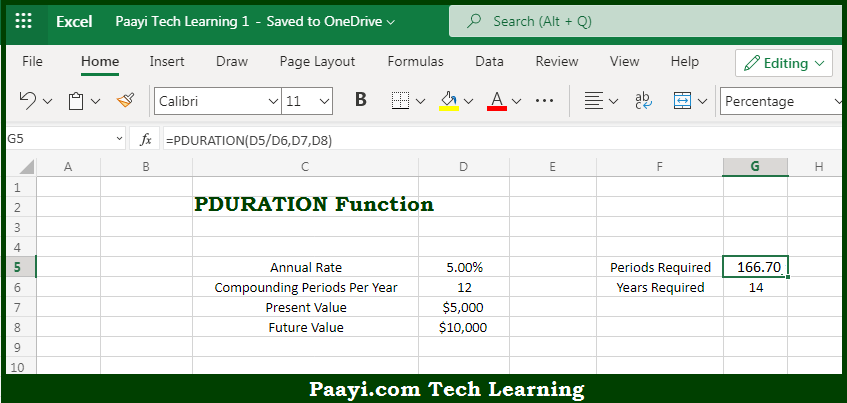# Learn How to Use Microsoft Excel PDURATION Function

Written by | 0 Comments | 544 Views

In this article, you will learn how to use the Microsoft Excel PDURATION function and its prime function in Microsoft Excel. You will also get to know the Microsoft Excel PDURATION function return value and syntax with the help of some examples.

Microsoft Excel PDURATION Function

The main purpose of the Microsoft Excel PDURATION function is to get the period required to reach the given value. That implies, with the help of PDURATION function you can able to return the number of periods required for an investment to reach a required value. So, with the help of PDURATION function, you can able to get the period required to reach the given value.

Return Value of PDURATION Function

The return value will be the required time in periods.

Syntax of PDURATION Function

=PDURATION(rate, pv, fv)

Where the arguments:

• rate - This is the interest rate of each period.
• pv - This is the present value of an investment.
• fv - This is the future value of an investment.

How to Use Microsoft Excel PDURATION Function?So we know that Microsoft Excel PDURATION function you can able to get the period required to reach the given value. That implies, with the help of the PDURATION function you can able to return the number of periods required for an investment to reach a required value. So, with the help of the PDURATION function, you can able to get the period required to reach the given value.